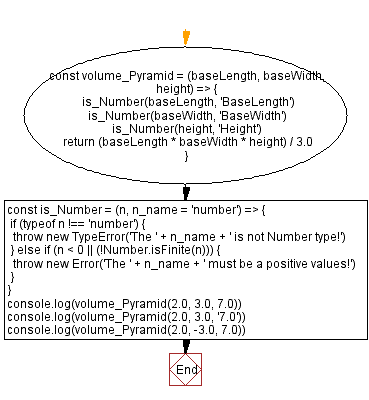# JavaScript: Volume of a Pyramid

## JavaScript Math: Exercise-59 with Solution

Write a JavaScript program to calculate the volume of a Pyramid.

In geometry, a pyramid is a polyhedron formed by connecting a polygonal base and a point, called the apex. Each base edge and apex form a triangle, called a lateral face. It is a conic solid with polygonal base. A pyramid with an n-sided base has n + 1 vertices, n + 1 faces, and 2n edges. All pyramids are self-dual.

Sample Solution:

HTML Code:

``````<!DOCTYPE html>
<html>
<meta charset="utf-8">
<title>JavaScript function to Volume of a Pyramid</title>
<body>

</body>
</html>
```
```

JavaScript Code:

``````const volume_Pyramid = (baseLength, baseWidth, height) => {
is_Number(baseLength, 'BaseLength')
is_Number(baseWidth, 'BaseWidth')
is_Number(height, 'Height')
return (baseLength * baseWidth * height) / 3.0
}
const is_Number = (n, n_name = 'number') => {
if (typeof n !== 'number') {
throw new TypeError('The ' + n_name + ' is not Number type!')
} else if (n < 0 || (!Number.isFinite(n))) {
throw new Error('The ' + n_name + ' must be a positive values!')
}
}
console.log(volume_Pyramid(2.0, 3.0, 7.0))
console.log(volume_Pyramid(2.0, 3.0, '7.0'))
console.log(volume_Pyramid(2.0, -3.0, 7.0))
```
```

Sample Output:

```14
--------------------------------------------------
Uncaught TypeError: The Height is not Number type!
at https://cdpn.io/cpe/boomboom/pen.js?key=pen.js-968300e8-d91d-a969-99b2-b16641f784d8:9
-------------------------------------------------------------------------------------------
Uncaught Error: The BaseWidth must be a positive values!
at https://cdpn.io/cpe/boomboom/pen.js?key=pen.js-6c222a2a-2e46-07e7-74dd-cd13634303e6:11
```

Flowchart:Live Demo:

See the Pen javascript-math-exercise-59 by w3resource (@w3resource) on CodePen.

Improve this sample solution and post your code through Disqus

Previous: Volume of a Cone.
Next: Volume of a Cylinder

What is the difficulty level of this exercise?

Test your Programming skills with w3resource's quiz.

﻿